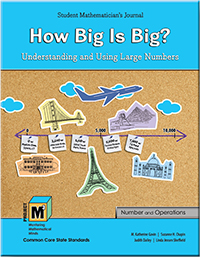# Project M³ Curriculum Units — Level 3-4

## How Big Is Big? Understanding and Using Large NumbersIn the National Research Council’s publication How Students Learn (2005), Sharon Griffin proposes that mathematics is not about numbers but rather about quantity, and numbers express those quantities. In her work with young children, she has found that numbers only acquire meaning for children when they realize that each number refers to a particular quantity. Often young children can singsong the numbers from 1 to 10 or even higher, but do not understand what each number represents. The same is true for very large numbers, not only for children but also for adults. It is really hard to picture what one hundred thousand looks like, let alone what one million or one billion look like. In this unit, students are introduced to numbers from 1,000 to 1,000,000 using a variety of models. The first chapter focuses on reading and writing large numbers, ordering numbers on a number line, and comparing numbers. Students apply their previous understanding of place value and extend their learning of the relative size of numbers in each place up to one million.

They create a physical model of 100,000 using base-ten unit cubes by first constructing a physical representation that occupies 10,000 units (a large space in itself) and then generating a new physical representation that is ten times bigger. This physical representation of 100,000 and how much bigger it is than the model of 10,000 demonstrates the exponential growth of place values in our system. Students also explore another physical model that depicts the ordering of large numbers: the number line. Creating number lines helps students gain a deeper understanding of the placement of numbers on the line and the appropriate spacing between numbers. Students then begin to use operations with large numbers: addition, subtraction, multiplication, and division. They build on their understanding of these operations using strategies that have helped them in the past to estimate answers as well as to find exact answers. They will also have opportunities to use the standard algorithms.

In Chapter 2, students continue to work with operations, in particular addition and multiplication. Throughout the chapter, students identify patterns in numbers, explain why these patterns occur, and learn how to use these patterns to help them compute. Students are introduced to patterns found in palindromes, numbers that read the same forwards and backwards such as 12,321. Students also examine multiplication patterns as they solve puzzles in which digits are missing from complex multiplication problems. Most of the problems require students to predict the outcomes two or three steps ahead and make modifications based on the different outcomes. They are constantly testing strategies, seeing if they work, and then revising to obtain the correct solution. In effect, they are working in the same manner as mathematicians. Finally, students investigate Fermi problems, real-life applications involving very large numbers such as “How many turkeys are eaten on Thanksgiving throughout the United States?” Students need to estimate and then extrapolate to find the answer.